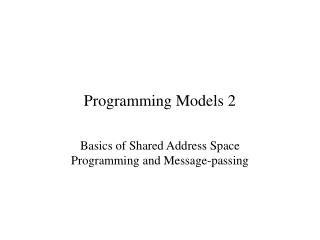DownloadDownload PresentationProgramming Models 2

# Programming Models 2

Télécharger la présentation## Programming Models 2

- - - - - - - - - - - - - - - - - - - - - - - - - - - E N D - - - - - - - - - - - - - - - - - - - - - - - - - - -
##### Presentation Transcript

1. Programming Models 2 Basics of Shared Address Space Programming and Message-passing

2. Shared Address Space Model • All memory is accessible to all processes • Processes are mapped to processors, typically by a symmetric OS • Coordination among processes: • by sharing variables • Avoid “stepping on toes”: • using locks and barriers

3. Matrix multiplication for (i=0; i<M; i++) for (j=0; j<N; j++) for (k=0; k<L; k++) C[i][j] += A[i][k]*B[k][j]; In a shared memory style, this program is trivial to parallelize Just have each processor deal with a different range of I (or J?) (or Both?)

4. SAS version: pseudocode size= M/numPEs( ); myStart = myPE( ) for (i=myStart; i<myStart+size; i++) for (j=0; j<N; j++) for (k=0; k<L; k++) C[i][j] += A[i][k]*B[k][j];

5. Running Example: computing pi • Area of circle : π*r*r • Ratio of the area of a circle, and that of the enclosing square: • π/4 • Method: compute a set of random number pairs (in the range 0-1) and count the number of pairs that fall inside the circle • The ratio gives us an estimate for π/4 • In parallel: Let each processor compute a different set of random number pairs (in the range 0-1) and count the number of pairs that fall inside the circle

6. Pi on shared memory int count; Lock countLock; piFunction(int myProcessor) { seed s = makeSeed(myProcessor); for (I=0; I<100000/P; I++) { x = random(s); y = random(s); if (x*x + y*y < 1.0) { lock(countLock);count++;unlock(countLock); }} barrier(); if (myProcessor == 0) { printf(“pi=%f\n”, 4*count/100000); }

7. main() { countLock = createLock(); parallel(piFunction); } The system needs to provide the functions for locks, barriers, and thread (or process) creation.

8. Pi on shared memory: efficient version int count; Lock countLock; piFunction(int myProcessor) { int c; seed s = makeSeed(myProcessor); for (I=0; I<100000/P; I++) { x = random(s); y = random(s); if (x*x + y*y < 1.0) c++; }} lock(countLock);count += c;;unlock(countLock); barrier(); if (myProcessor == 0) { printf(“pi=%f\n”, 4*count/100000); }

9. Real SAS systems • Posix threads (Pthreads) is a standard for threads-based shared memory programming • Shared memory calls: just a few, normally standard calls • In addition, lower level calls: fetch-and-inc, fetch-and-add

10. Message Passing • Assume that processors have direct access to only their memory • Each processor typically executes the same executable, but may be running different part of the program at a time

11. Message passing basics: • Basic calls: send and recv • send(int proc, int tag, int size, char *buf); • recv(int proc, int tag, int size, char * buf); • Recv may return the actual number of bytes received in some systems • tag and proc may be wildcarded in a recv: • recv(ANY, ANY, 1000, &buf); • broadcast: • Other global operations (reductions)